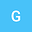The linearized Poisson-Nernst-Planck system as heat flow on the interval under non-local boundary conditions
•## Abstract

The linearized of the Poisson-Nernst-Planck (PNP) equation under closed ends around a neutral state is studied. It is reduced to a damped heat equation under non-local boundary conditions, which leads to a stochastic interpretation of the linearized equation as a Brownian particle which jump and is reflected, at Poisson distributed time, to one of the end points of the channel, with a probability which is proportional to its distance from this end point. An explicit expansion of the heat kernel reveals the eigenvalues and eigenstates of both the PNP equation and its adjoint. For this, we take advantage of the representation of the resulvent operator and recover the heat kernel by applying the inverse Laplace transform.
17 Mar 2023Submitted to Mathematical Methods in the Applied Sciences
20 Mar 2023Submission Checks Completed
20 Mar 2023Assigned to Editor
31 Mar 2023Review(s) Completed, Editorial Evaluation Pending
06 Apr 2023Reviewer(s) Assigned
12 Jun 2023Editorial Decision: Revise Minor